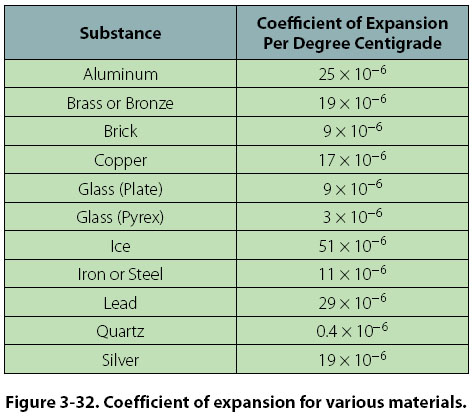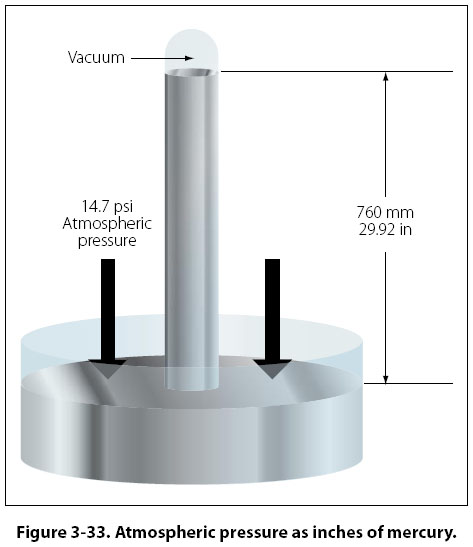Thermal Expansion/Contraction Thermal expansion takes place in solids, liquids, and gases when they are heated. With few exceptions, solids will expand when heated and contract when cooled. Because the molecules of solids are much closer together and are more strongly attracted to each other, the expansion of solids when heated is very slight in comparison to the expansion in liquids and gases. The expansion of fluids is discussed in the study of Boyle’s law. Thermal expansion in solids must be explained in some detail because of its close relationship to aircraft metals and materials. Because some substances expand more than others, it is necessary to measure experimentally the exact rate of expansion of each one. The amount that a unit length of any substance expands for a one degree rise in temperature is known as the coefficient of linear expansion for that substance. The coefficient of linear expansion for various materials is shown in Figure 3-32.To estimate the expansion of any object, such as a steel rail, it is necessary to know three things about it: its length, the rise in temperature to which it is subjected, and its coefficient of expansion. This relationship is expressed by the equation: Expansion = (coefficient) × (length) × (rise in temperature) If a steel rod measures exactly 9 ft at 21°C, what is its length at 55°C? The coefficient of expansion for steel is 11 × 10-6. Expansion = (11 × 10-6) × (9 feet) × 34° Expansion = 0.003366 feet This amount, when added to the original length of the rod, makes the rod 9.003366 ft long. Its length has only increased by 4/100 of an inch. The increase in the length of the rod is relatively small, but if the rod were placed where it could not expand freely, there would be a tremendous force exerted due to thermal expansion. Thus, thermal expansion must be taken into consideration when designing airframes, powerplants, or related equipment. Pressure Pressure is the amount of force acting on a specific amount of surface area. The force is typically measured in pounds and the surface area in square inches, making the units of pressure pounds per square inch or psi. If a 100-lb weight was placed on top of a block with a surface area of 10 in2, the average weight distribution would be 10 lb for each of the square inches (100 ÷ 10), or 10 psi. When atmospheric pressure is being measured, in addition to psi, other means of pressure measurement can be used. These include inches or millimeters of mercury, and millibars. Standard day atmospheric pressure is equal to 14.7 psi, 29.92 inches of mercury ("Hg), 760 millimeters of mercury (mm Hg), or 1013.2 millibars. The relationship between these units of measure is as follows: 1 psi = 2.04 "Hg 1 psi = 51.7 mm Hg 1 psi = 68.9 millibars The concept behind measuring pressure in inches of mercury involves filling a test tube with the liquid mercury and then covering the top. The test tube is then turned upside down and placed in an open container of mercury, and the top is uncovered. Gravity acting on the mercury in the test tube will try to make the mercury run out. Atmospheric pressure pushing down on the mercury in the open container tries to make the mercury stay in the test tube. At some point these two forces (gravity and atmospheric pressure) will equal out and the mercury will stabilize at a certain height in the test tube. Under standard day atmospheric conditions, the air in a 1-in2 column extending all the way to the top of the atmosphere would weigh 14.7 lb. A 1 in2 column of mercury, 29.92 inches tall, would also weigh 14.7 lb. That is why 14.7 psi is equal to 29.92 "Hg. Figure 3-33 demonstrates this point.©AvStop Online Magazine                                                                                                                                                      Contact Us              Return To Books